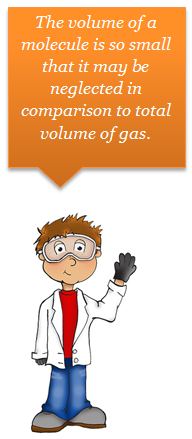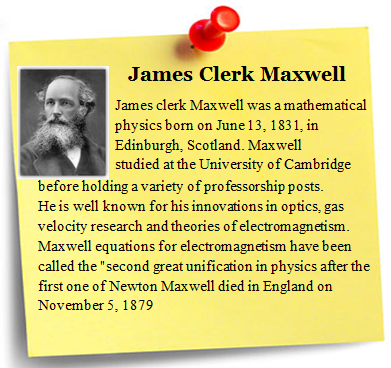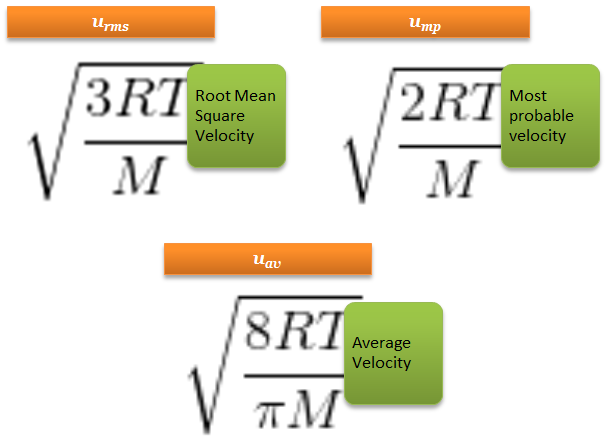×#### Thank you for registering.

One of our academic counsellors will contact you within 1 working day.

Click to Chat

1800-1023-196

+91-120-4616500

CART 0

• 0

MY CART (5)

Use Coupon: CART20 and get 20% off on all online Study Material

ITEM
DETAILS
MRP
DISCOUNT
FINAL PRICE
Total Price: Rs.

There are no items in this cart.
Continue ShoppingKinetic Molecular Theory of Gases

Table of Content

Postulates of Kinetic Molecular Theory

The Kinetic Equation

Velocities of Gas Molecules

Kinetic Energy of Gas

Related ResourcesThe various gas laws which we have studied so far were based an experimental facts. The theory that provides an explanation for the various experimental observations about a gas is based on the Kinetic Molecular model. It is assumed that all gases are made up of identical molecules, which are in a state of constant random motion.

Postulates of Kinetic Molecular Theory

Maxwell proposed the following postulates under the heading of kinetic theory of gases.Each gas is made up of a large number of small (tiny) particles known as molecules.

The volume of a molecule is so small that it may be neglected in comparison to total volume of gas.

The molecules are never in stationary state but they are believed to be in chaotic (random) motion. They travel in straight line in all possible directions with altogether different but constant velocities. The direction of motion is changed by the collision with container or with other molecules.

The molecules are perfectly elastic and bear no change in energy during collision.

The effect of gravity on molecular motion is negligible.

The temperature of gas is a measure of its kinetic energy.

The pressure of the gas is thus due to the continuous collision of molecules on the walls of container. Pressure P = Force/Area.

Refer to the following video for kinetic molecular theories of gases

The Kinetic EquationMaxwell also derived an equation on the basis of above assumption as

Where P = Pressure of gas

V = Volume of gas

m = mass of one molecule  of gas

n = no. of molecules of gas

u = root mean square velocity of molecules

For 1 mole n = N is Avogadro number

m  N = Molecular mass M

For 1 mole of an Ideal gas, pV = RT

so,

Velocities of Gas MoleculesAverage Velocity

As per kinetic theory of gases, each molecule is moving with altogether different velocity. Let ‘n’ molecules be present in a given mass of gas, each one moving with velocity v1, v2, v3… vn.

The average velocity or UaV = average of all such velocity terms.

Average velocity =

Root Mean Square Velocity:-

Maxwell proposed the term Urms as the square root of means of square of all such velocities.

also

Most probable velocity:-

It is the velocity which is possessed by maximum no. of molecules.

Furthermore

Kinetic Energy of Gas

As per kinetic equation

For 1 mole m × n = Molecular Mass (M)

Also

Where k is the Boltzmann constant (k = R / N)

Solved Examples

Question 1:

Calculate rms speed of O2 at 273 K and 1 × 105Pa pressure. The density of O2under these conditions is 1.42 kg m–3.

Solution:

Data are given in SI units

C = √3P / d = √3 × 105 / 1.42 = 459.63 m sec–1

__________________________________________________

Question 2:

At what temperature will the r.m.s. velocity of oxygen be one and half times of its value at N.T.P.?

Solution:

1/2 mc2 = 3/2 KT

Suppose the temperature required is T¢ when the velocity will be 3/2 C

3/2C / C = √T'/T or, T' = 9/4 × 273 = 614.25°K

_____________________________________________

Question 3:

Calculate K.E. of 4g of N2 at – 13°C.

Solution:

EK = 3/2 nRT = 3/2 × 4/28 × 8.314 × 260  = 463.21 J mol–1Question 1: Which of the following statements of kinetic molecular theory is incorrect?

a. The effect of gravity reduced the molecular motion .

b. The temperature of gas is a measure of its kinetic energy. KE µ absolute temperature.

c. The pressure of the gas is thus due to the continuous collision of molecules on the walls of container

d. The volume of a molecule is so small that it may be neglected in comparison to total volume of gas.

Question 2: In the equation

u2 represents

a. average velocity of molecules.

b. root mean square velocity of molecules.

c. most probable velocity of molecules.

d. final velocity of molecules.

Question 3: Kinetic energy of gas is given by

a. 3/2 nRT

b.1/2 nRT

c. 3/2 nKT

d. 1/2 nRT

Question 4:  The velocity which is possessed by maximum no. of molecules is called

a. average velocity.

b. root mean square velocity.

c. most probable velocity.

d. escape velocity.Q.1

Q.2

Q.3

Q.4

a

b

a

c

Related Resources

Look here for Reference books for IIT JEE Chemistry

You can also refer States of matter

To read more, Buy study materials of States of Matter comprising study notes, revision notes, video lectures, previous year solved questions etc. Also browse for more study materials on Chemistry here.### Course Features

• 731 Video Lectures
• Revision Notes
• Previous Year Papers
• Mind Map
• Study Planner
• NCERT Solutions
• Discussion Forum
• Test paper with Video Solution